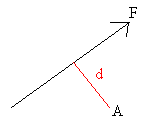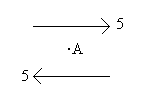## Title

Moments
Quick revise

The moment of a force about a point is found by multiplying the magnitude of the force by the perpendicular distance from the point to the line of action of the force.• The moment of the force F about point A is F × d.

Moments can be either clockwise or anti-clockwise. In the above example, the moment is clockwise (because the force would cause a body to move in a clockwise direction relative to A).

The moment of a force about a point measures the turning effect of the force about that point.

Couples

A couple is a system of forces which has a net moment but does not have a resultant force in any one direction.

Here is a very simple couple:In any direction, the resultant force is zero but the moment about A is clearly not zero. Such forces acting on a body might cause the body to rotate, but not move in any one direction.

If a system is in equilibrium, the system will have zero resultant force and the sum of the moments about any point will be zero.

Rate: